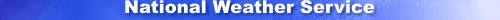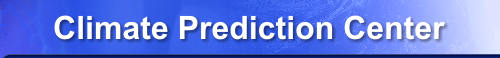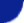Site Map News Organization SearchHOME > Monitoring_and_Data > Oceanic and Atmospheric Data > Reanalysis: Atmospheric Data > wgrib2-Set_len

Introduction

Grib2 messages (records) are comprised of 9 sections (0-8). Sections 0 and 8 are 16 and 4 bytes long, respectively. When the message contains submessages, each submessage contains 9 sections but some of the sections can be shared with the other submessages. The -Set_late option shows the length of each section except for sections 0 and 8.

-Sec_len

Examples

\$ wgrib2 small.grb2 -Sec_len
1:0:Sec size msg=188 id(1)=21 local(2)=0 grid(3)=72 product(4)=37 data-rep(5)=21 bitmap(6)=6 data(7)=11

The size of the grib message is 188 bytes.
Section 1 is 21
Section 2 is missing and not used
Section 3 is 72
Section 4 is 37
Section 5 is 21
Section 6 is 6
Section 7 is 11